# RD Sharma Solutions for Class 11 Chapter 32 - Statistics Exercise 32.2

Here in this exercise, we shall discuss mean deviation of a discrete frequency distribution, along with an algorithm to calculate the mean deviation. Students who find difficulty in solving maths problems can follow the solutions prepared by the experts, who have formulated the solutions in the simplest way, for any student to understand easily. The main aim of preparing solutions is to help students solve textbook problems without any difficulty. Students who are not able to clear doubts during class hours can clear them by referring to the RD Sharma Class 11 Maths Solutions. Students can effortlessly download the solutions pdf, from the links given below.

## Download the pdf of RD Sharma Solutions for Class 11 Maths Exercise 32.2 Chapter 32 – Statistics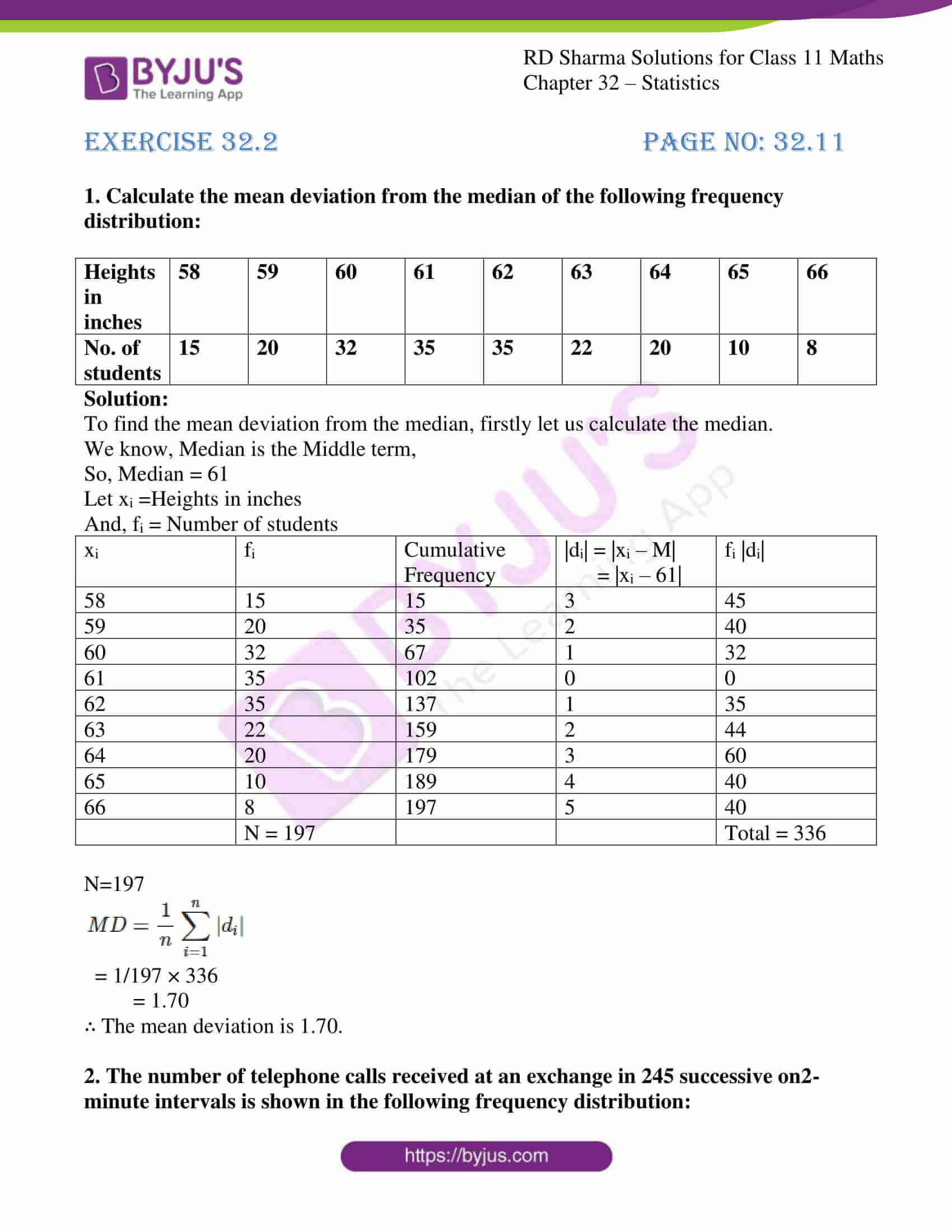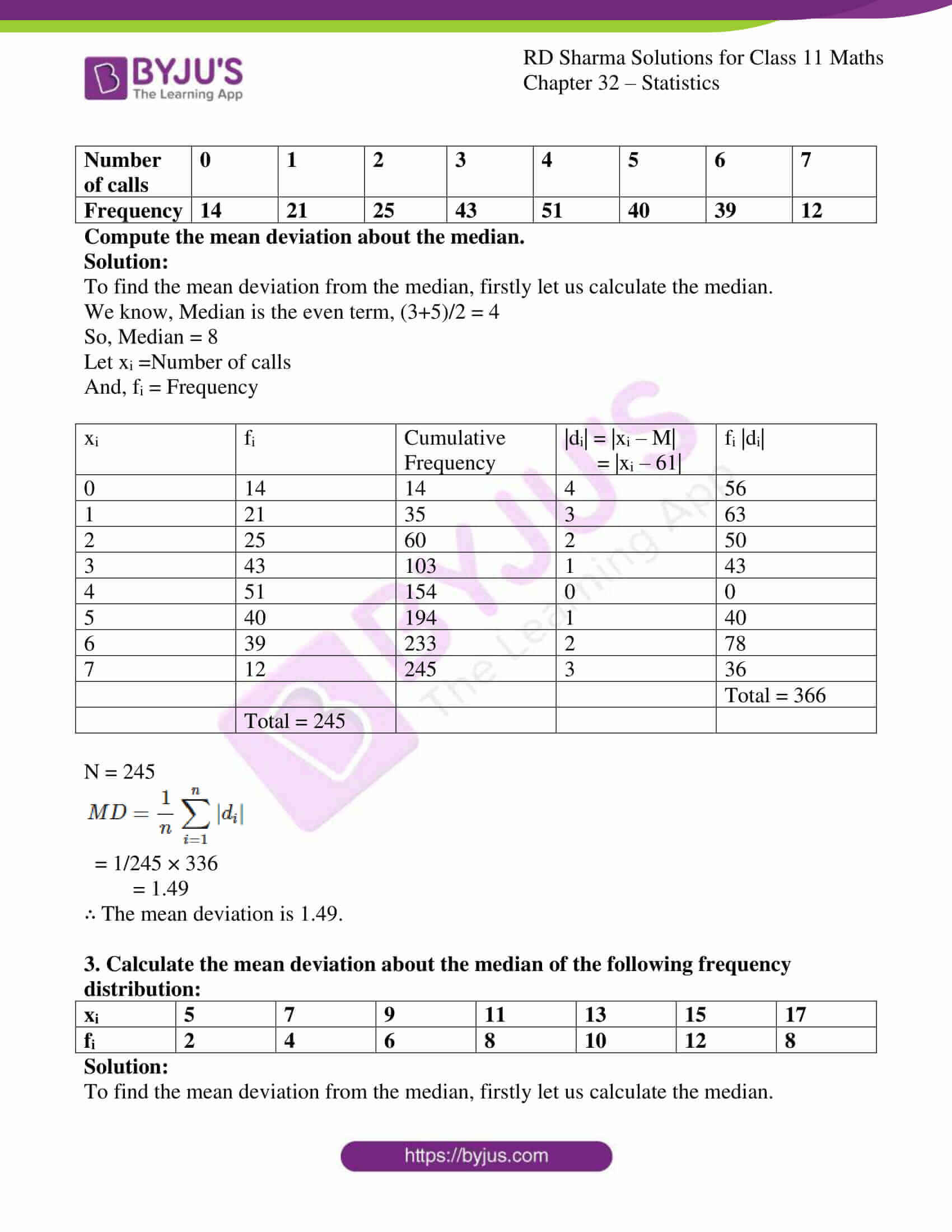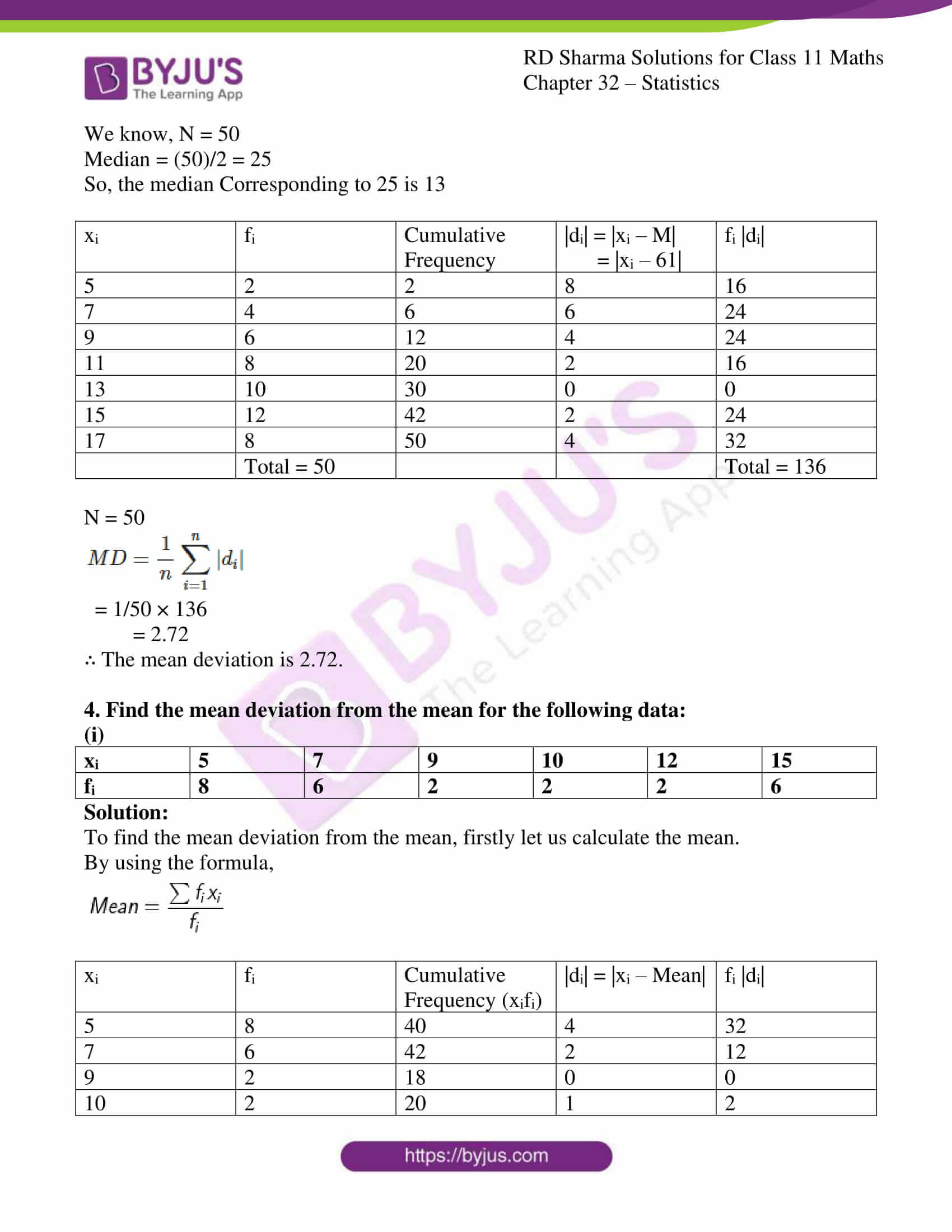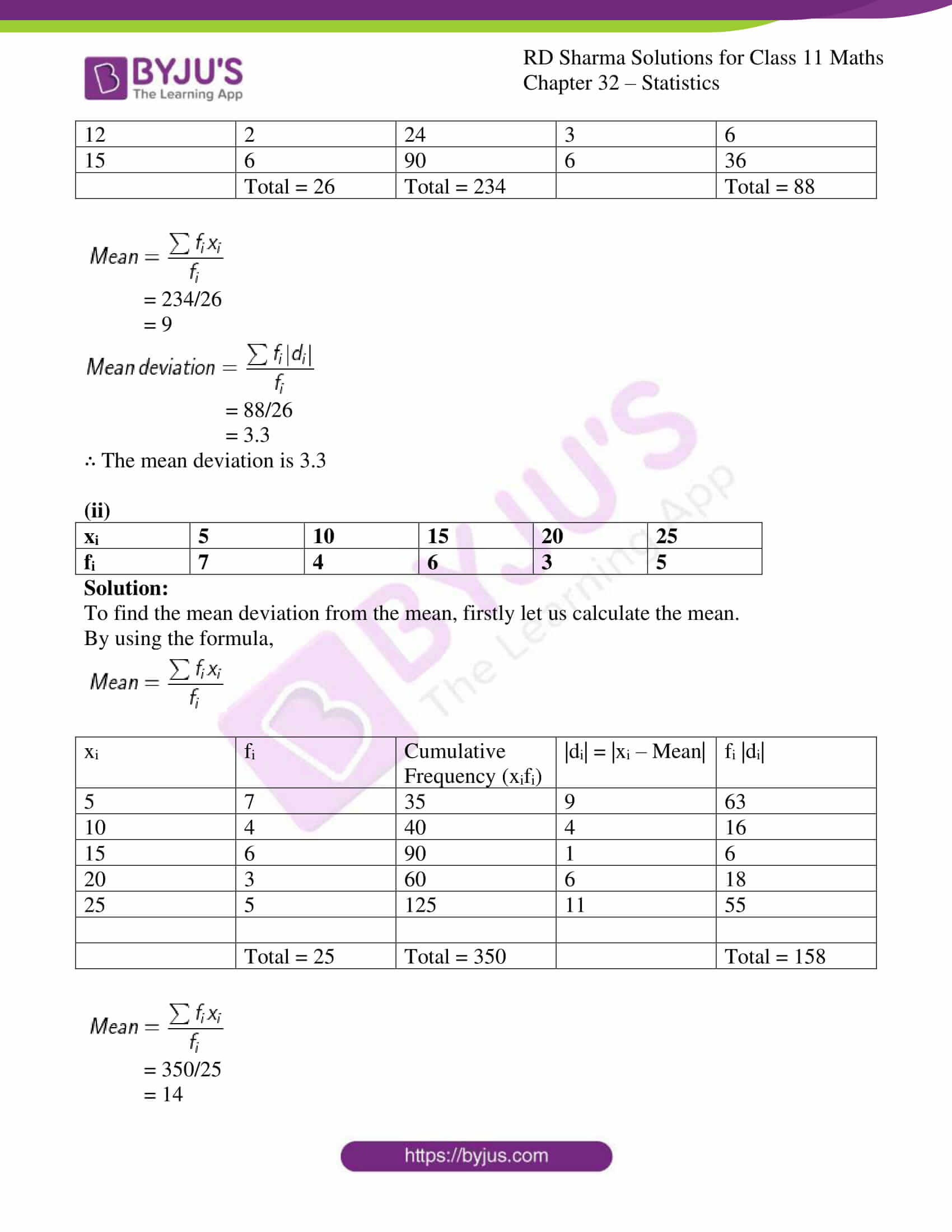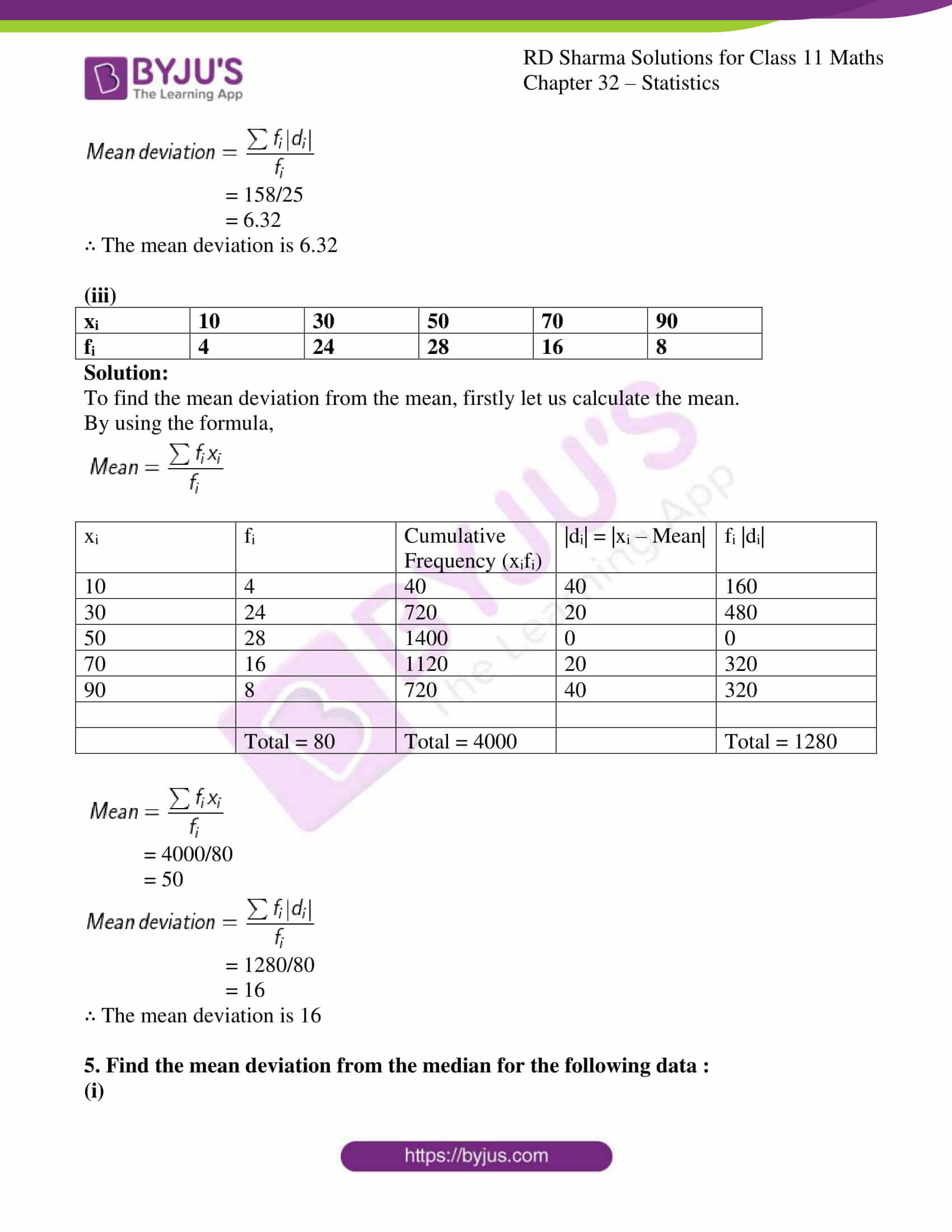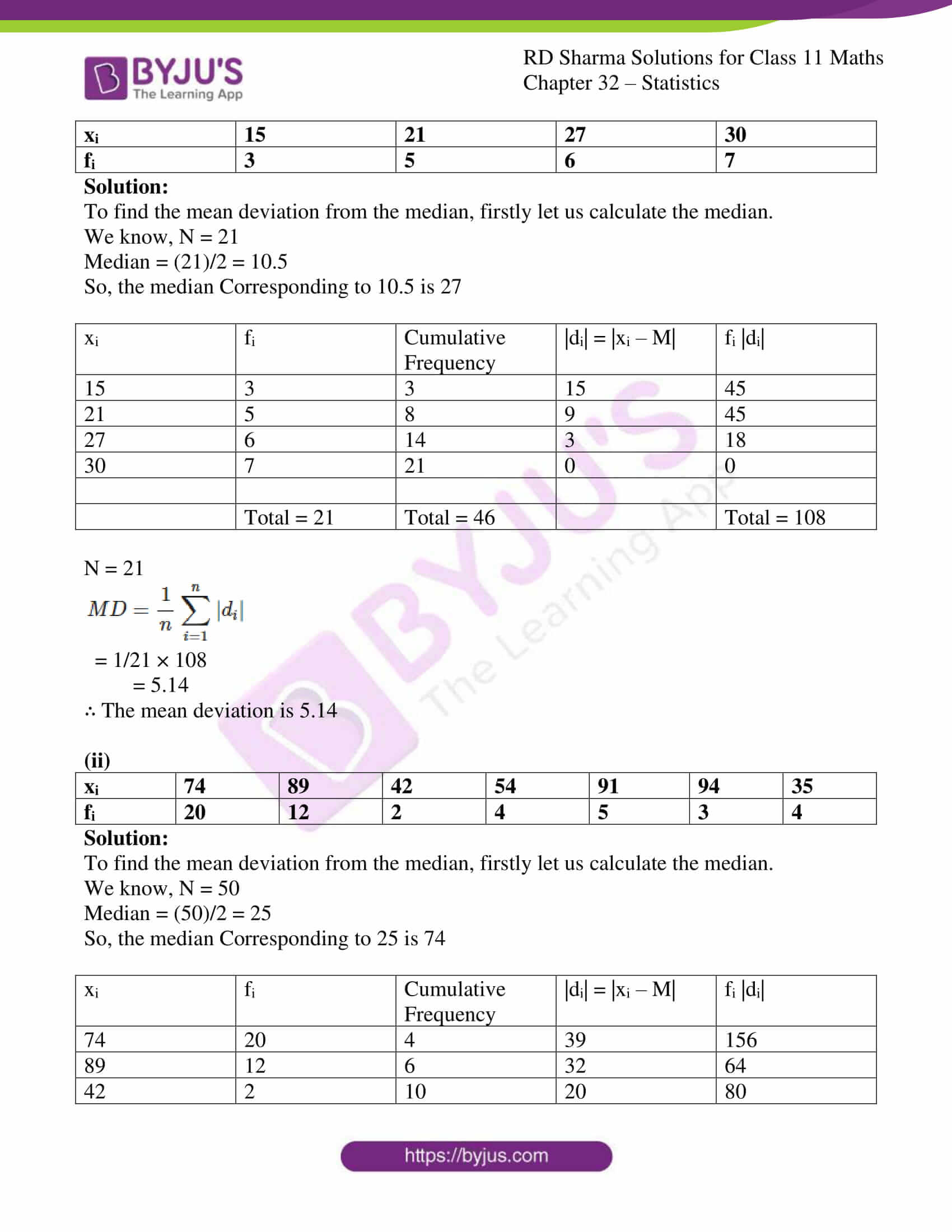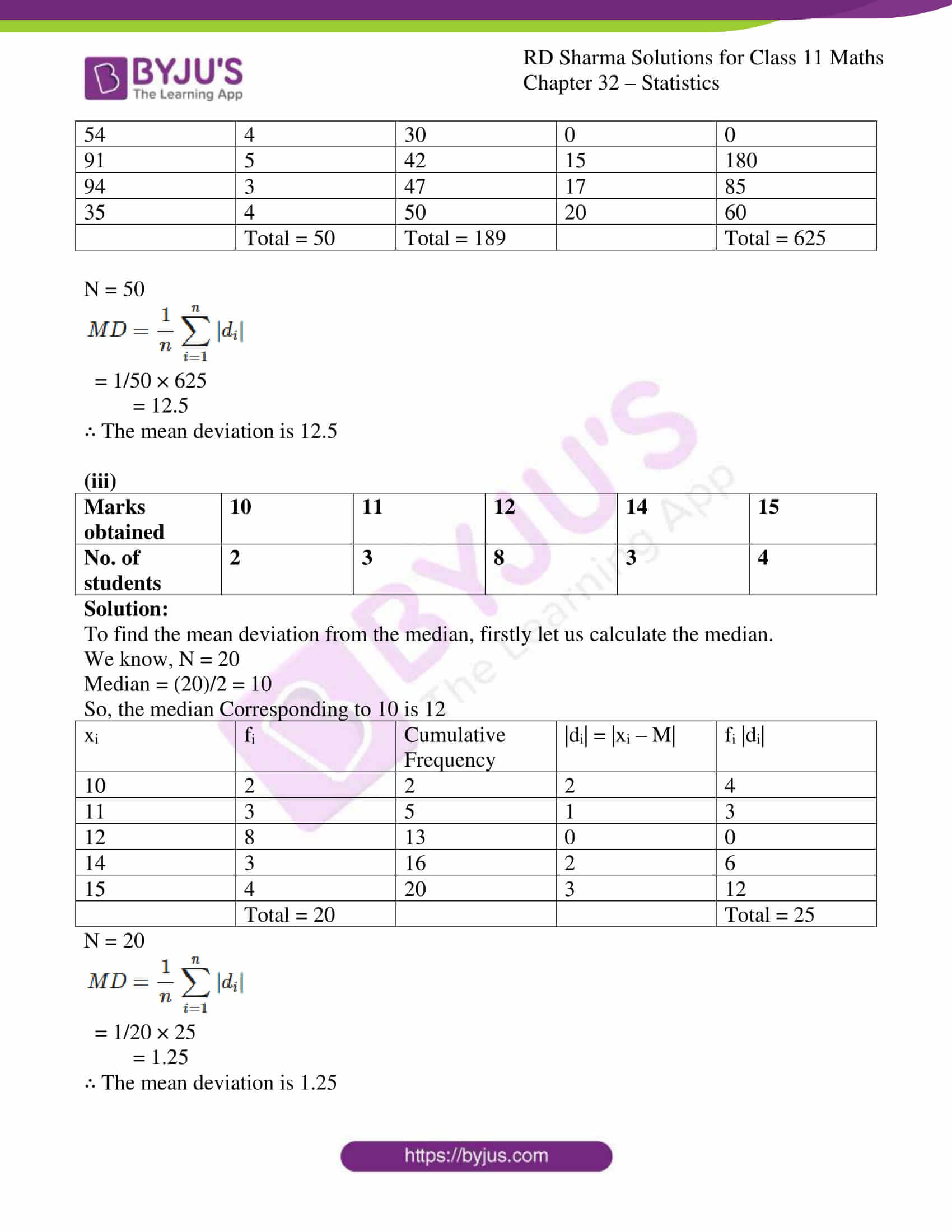### Access answers to RD Sharma Solutions for Class 11 Maths Exercise 32.2 Chapter 32 – Statistics

#### EXERCISE 32.2 PAGE NO: 32.11

1. Calculate the mean deviation from the median of the following frequency distribution:

 Heights in inches 58 59 60 61 62 63 64 65 66 No. of students 15 20 32 35 35 22 20 10 8

Solution:

To find the mean deviation from the median, firstly let us calculate the median.

We know, Median is the Middle term,

So, Median = 61

Let xi =Heights in inches

And, fi = Number of students

 xi fi Cumulative Frequency |di| = |xi – M| = |xi – 61| fi |di| 58 15 15 3 45 59 20 35 2 40 60 32 67 1 32 61 35 102 0 0 62 35 137 1 35 63 22 159 2 44 64 20 179 3 60 65 10 189 4 40 66 8 197 5 40 N = 197 Total = 336

N=197

$$MD = \frac{1}{n}\sum_{i=1}^{n}|d_{i}|$$

= 1/197 × 336

= 1.70

∴ The mean deviation is 1.70.

2. The number of telephone calls received at an exchange in 245 successive on2-minute intervals is shown in the following frequency distribution:

 Number of calls 0 1 2 3 4 5 6 7 Frequency 14 21 25 43 51 40 39 12

Compute the mean deviation about the median.

Solution:

To find the mean deviation from the median, firstly let us calculate the median.

We know, Median is the even term, (3+5)/2 = 4

So, Median = 8

Let xi =Number of calls

And, fi = Frequency

 xi fi Cumulative Frequency |di| = |xi – M| = |xi – 61| fi |di| 0 14 14 4 56 1 21 35 3 63 2 25 60 2 50 3 43 103 1 43 4 51 154 0 0 5 40 194 1 40 6 39 233 2 78 7 12 245 3 36 Total = 366 Total = 245

N = 245

$$MD = \frac{1}{n}\sum_{i=1}^{n}|d_{i}|$$

= 1/245 × 336

= 1.49

∴ The mean deviation is 1.49.

3. Calculate the mean deviation about the median of the following frequency distribution:

 xi 5 7 9 11 13 15 17 fi 2 4 6 8 10 12 8

Solution:

To find the mean deviation from the median, firstly let us calculate the median.

We know, N = 50

Median = (50)/2 = 25

So, the median Corresponding to 25 is 13

 xi fi Cumulative Frequency |di| = |xi – M| = |xi – 61| fi |di| 5 2 2 8 16 7 4 6 6 24 9 6 12 4 24 11 8 20 2 16 13 10 30 0 0 15 12 42 2 24 17 8 50 4 32 Total = 50 Total = 136

N = 50

$$MD = \frac{1}{n}\sum_{i=1}^{n}|d_{i}|$$

= 1/50 × 136

= 2.72

∴ The mean deviation is 2.72.

4. Find the mean deviation from the mean for the following data:

(i)

 xi 5 7 9 10 12 15 fi 8 6 2 2 2 6

Solution:

To find the mean deviation from the mean, firstly let us calculate the mean.

By using the formula,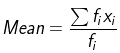xi fi Cumulative Frequency (xifi) |di| = |xi – Mean| fi |di| 5 8 40 4 32 7 6 42 2 12 9 2 18 0 0 10 2 20 1 2 12 2 24 3 6 15 6 90 6 36 Total = 26 Total = 234 Total = 88= 234/26

= 9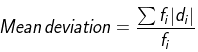= 88/26

= 3.3

∴ The mean deviation is 3.3

(ii)

 xi 5 10 15 20 25 fi 7 4 6 3 5

Solution:

To find the mean deviation from the mean, firstly let us calculate the mean.

By using the formula,xi fi Cumulative Frequency (xifi) |di| = |xi – Mean| fi |di| 5 7 35 9 63 10 4 40 4 16 15 6 90 1 6 20 3 60 6 18 25 5 125 11 55 Total = 25 Total = 350 Total = 158= 350/25

= 14= 158/25

= 6.32

∴ The mean deviation is 6.32

(iii)

 xi 10 30 50 70 90 fi 4 24 28 16 8

Solution:

To find the mean deviation from the mean, firstly let us calculate the mean.

By using the formula,xi fi Cumulative Frequency (xifi) |di| = |xi – Mean| fi |di| 10 4 40 40 160 30 24 720 20 480 50 28 1400 0 0 70 16 1120 20 320 90 8 720 40 320 Total = 80 Total = 4000 Total = 1280= 4000/80

= 50= 1280/80

= 16

∴ The mean deviation is 16

5. Find the mean deviation from the median for the following data :

(i)

 xi 15 21 27 30 fi 3 5 6 7

Solution:

To find the mean deviation from the median, firstly let us calculate the median.

We know, N = 21

Median = (21)/2 = 10.5

So, the median Corresponding to 10.5 is 27

 xi fi Cumulative Frequency |di| = |xi – M| fi |di| 15 3 3 15 45 21 5 8 9 45 27 6 14 3 18 30 7 21 0 0 Total = 21 Total = 46 Total = 108

N = 21

$$MD = \frac{1}{n}\sum_{i=1}^{n}|d_{i}|$$

= 1/21 × 108

= 5.14

∴ The mean deviation is 5.14

(ii)

 xi 74 89 42 54 91 94 35 fi 20 12 2 4 5 3 4

Solution:

To find the mean deviation from the median, firstly let us calculate the median.

We know, N = 50

Median = (50)/2 = 25

So, the median Corresponding to 25 is 74

 xi fi Cumulative Frequency |di| = |xi – M| fi |di| 74 20 4 39 156 89 12 6 32 64 42 2 10 20 80 54 4 30 0 0 91 5 42 15 180 94 3 47 17 85 35 4 50 20 60 Total = 50 Total = 189 Total = 625

N = 50

$$MD = \frac{1}{n}\sum_{i=1}^{n}|d_{i}|$$

= 1/50 × 625

= 12.5

∴ The mean deviation is 12.5

(iii)

 Marks obtained 10 11 12 14 15 No. of students 2 3 8 3 4

Solution:

To find the mean deviation from the median, firstly let us calculate the median.

We know, N = 20

Median = (20)/2 = 10

So, the median Corresponding to 10 is 12

 xi fi Cumulative Frequency |di| = |xi – M| fi |di| 10 2 2 2 4 11 3 5 1 3 12 8 13 0 0 14 3 16 2 6 15 4 20 3 12 Total = 20 Total = 25

N = 20

$$MD = \frac{1}{n}\sum_{i=1}^{n}|d_{i}|$$

= 1/20 × 25

= 1.25

∴ The mean deviation is 1.25